Ex 12.2

Chapter 12 Class 7 Symmetry
Serial order wise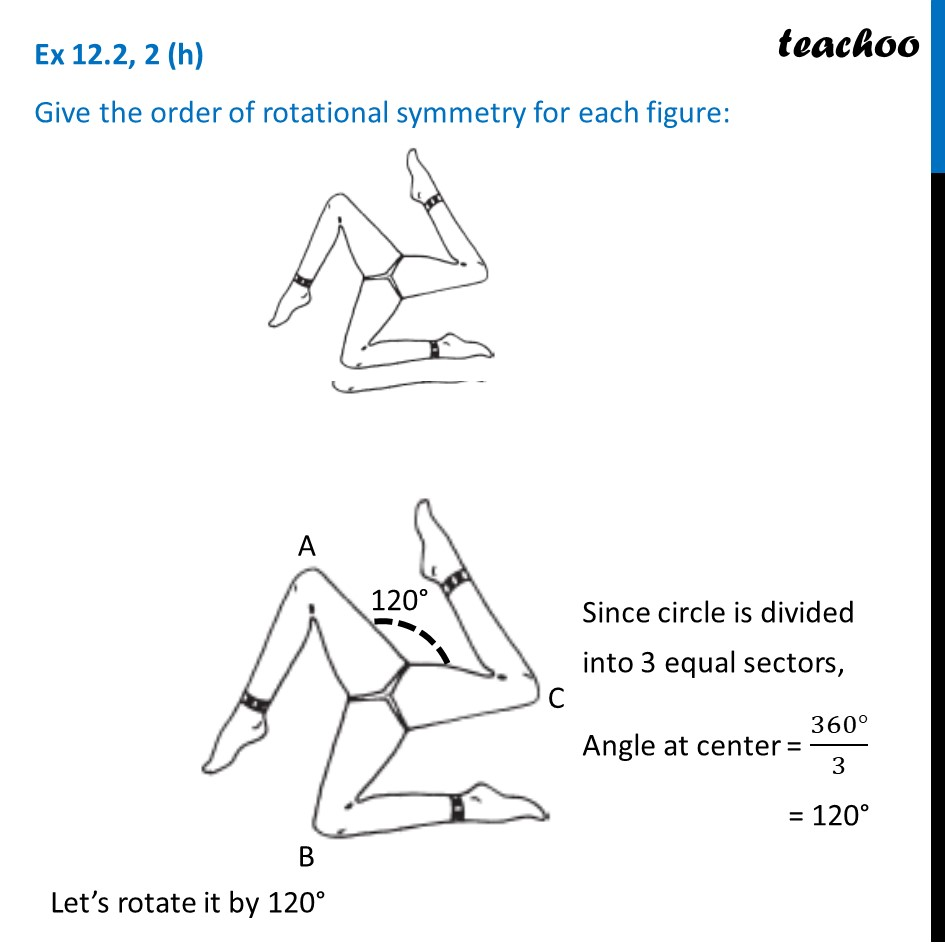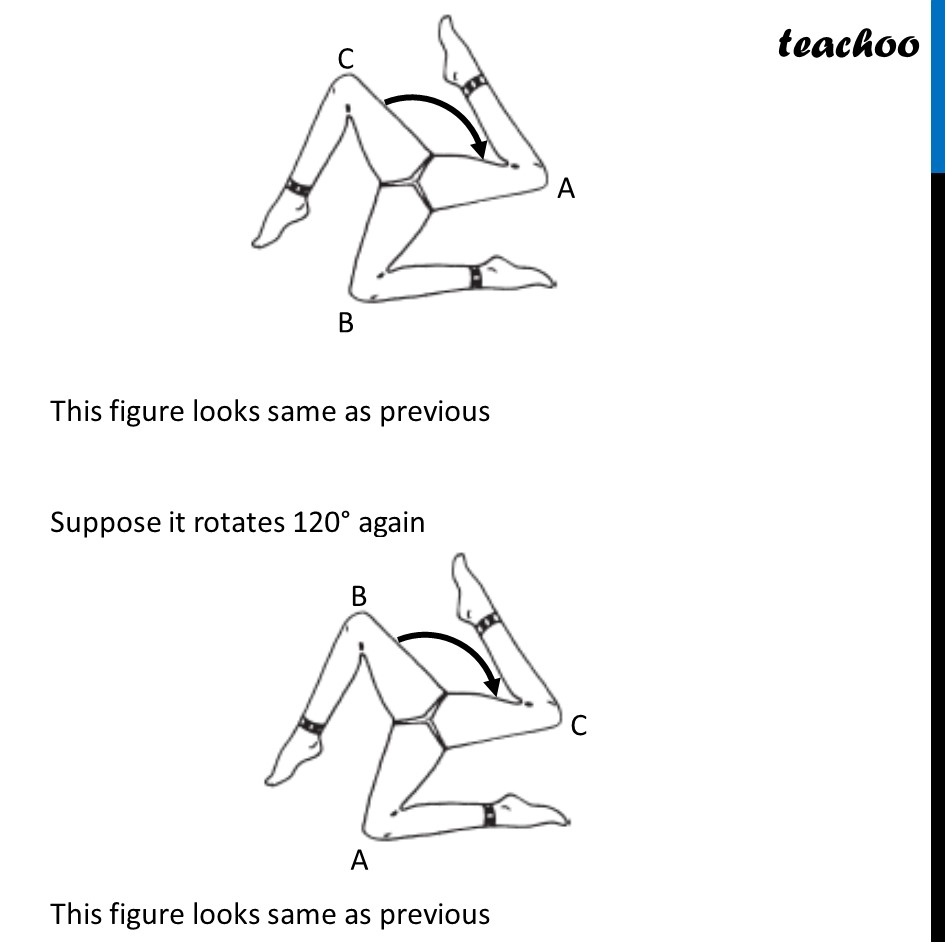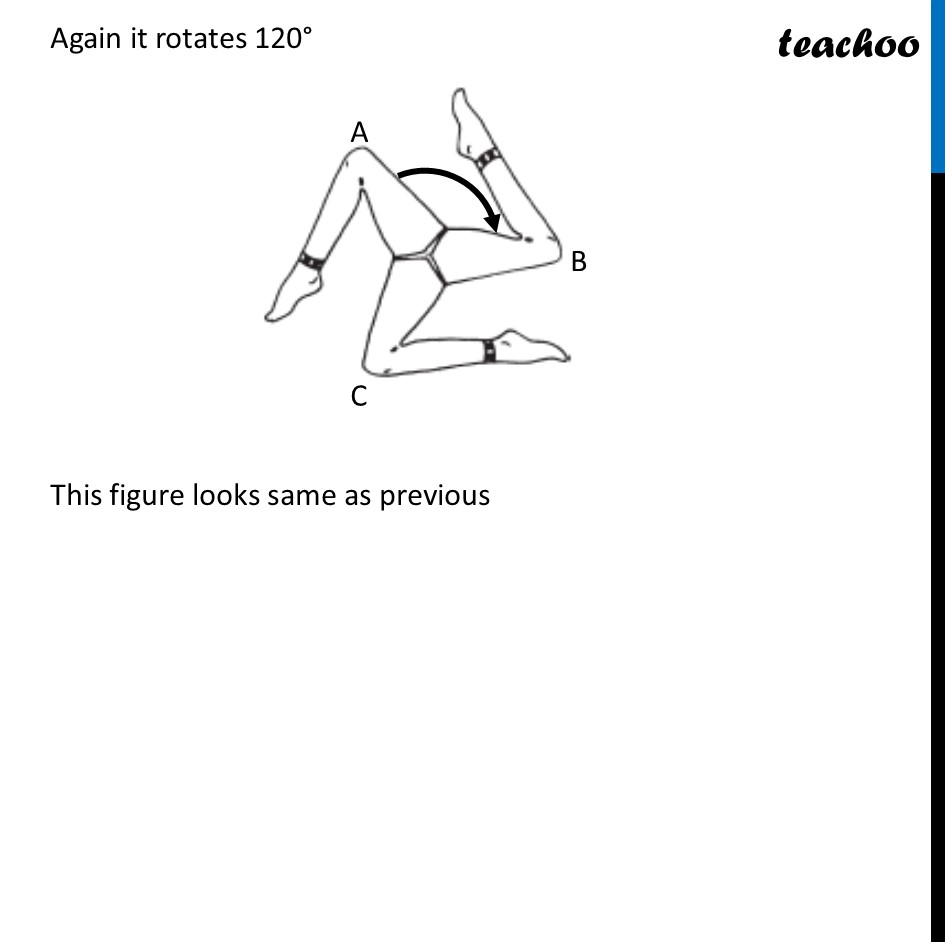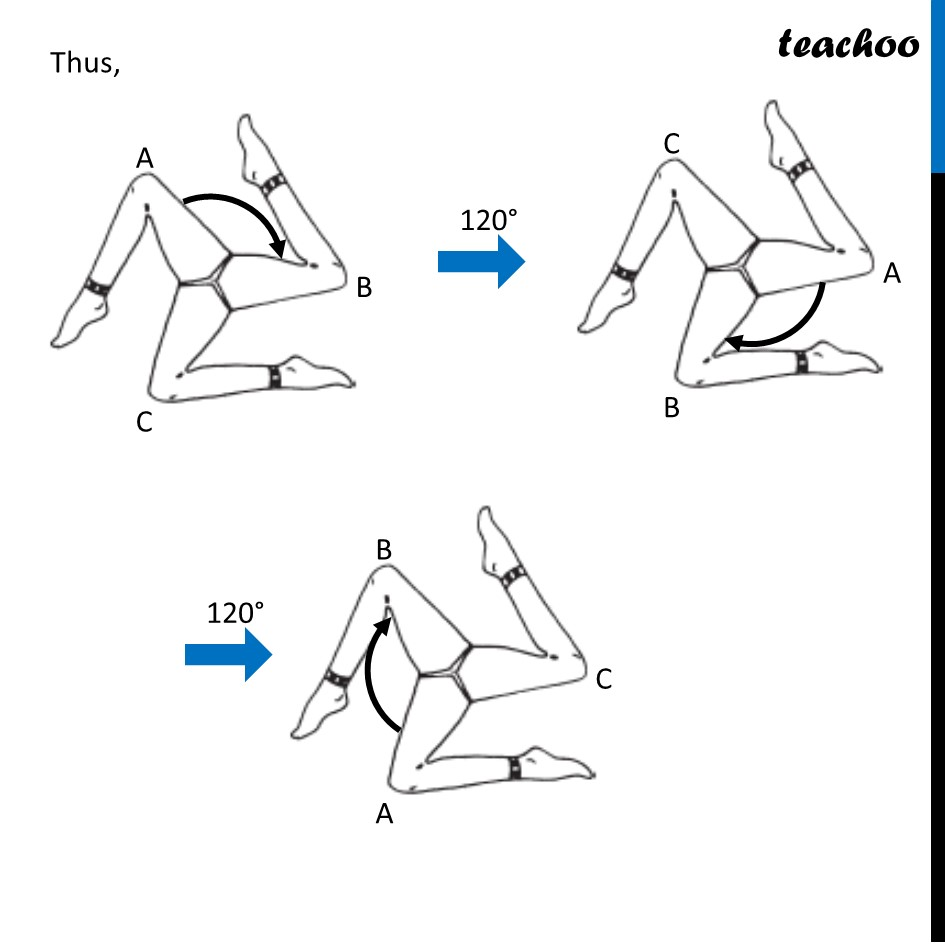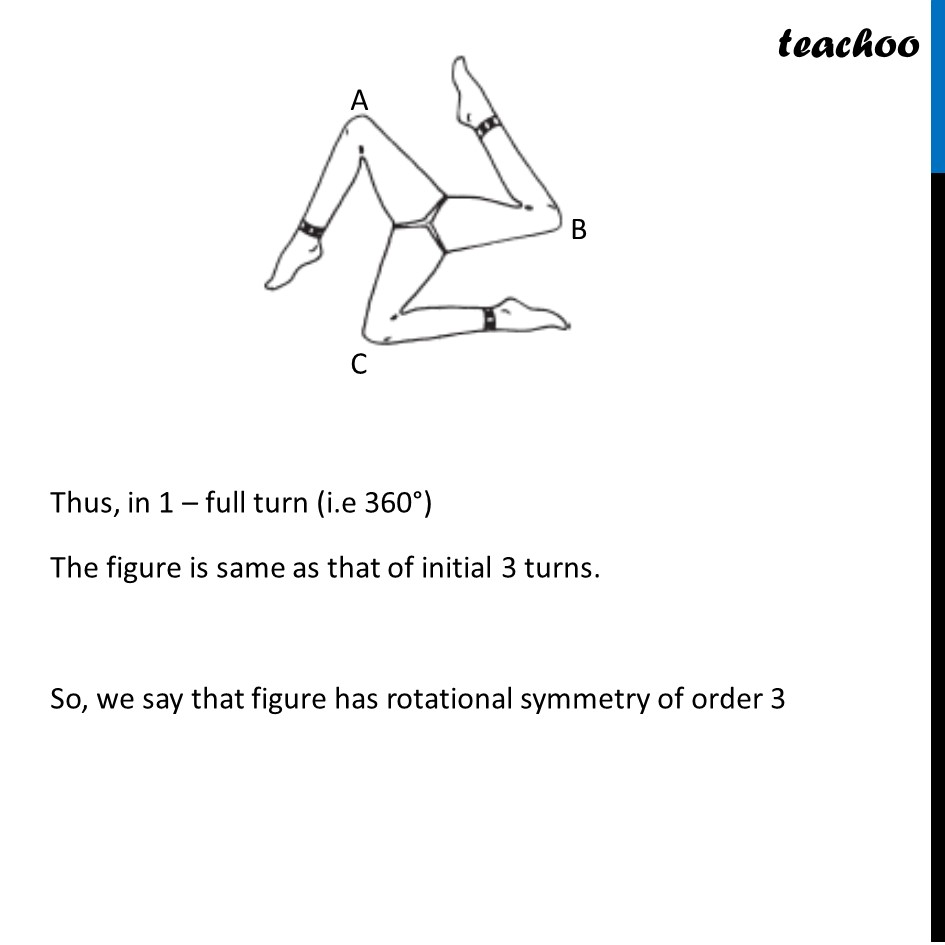Learn in your speed, with individual attention - Teachoo Maths 1-on-1 Class

### Transcript

Ex 12.2, 2 (h) Give the order of rotational symmetry for each figure: Since circle is divided into 3 equal sectors, Angle at center = (360°)/3 = 120° This figure looks same as previous Suppose it rotates 120° again This figure looks same as previous Thus, in 1 – full turn (i.e 360°) The figure is same as that of initial 3 turns. So, we say that figure has rotational symmetry of order 3